Supplementary

# Supplementary Materials #

This website maintains the supplementary materials related to the following paper:

Ke Li, Guiyu Lai+, Xin Yao, “Interactive Evolutionary Multi-Objective Optimization via Learning-to-Rank”, IEEE Trans. Evol. Comput., accepted for publication, 2022.

It consists of the following parts:

• The source code of this paper can be found from our Github repo.
• Appendix document of this paper can be found from this Dropbox link.
• Video clips of the best policy obtained by different algorithms. In particular, $$f_1$$ is the x-axis speed, $$f_2$$ is the y-axis speed, $$f_3 \text{ is the energy consumption}$$
• BC-EMO: $$(f_1=68.45730, f_2=111.72400, f_3=95.55980)^\top$$
• NEMO-0: $$(f_1=68.45730, f_2=111.72400, f_3=95.55980)^\top$$
• I-MOEA/D-PLVF: $$(f_1=156.62800, f_2=30.54620, f_3=75.83200)^\top$$
• IEMO/D: $$(f_1=66.09890, f_2=61.94570, f_3=75.91520)^\top$$
• I-NSGA-II/LTR: $$(f_1=100.52500, f_2=96.36920, f_3=100.34000)^\top$$
• I-MOEA/D/LTR: $$(f_1=114.31000, f_2=68.71040, f_3=102.49200)^\top$$
• I-R2-IBEA/LTR: $$(f_1=120.48400, f_2=74.36900, f_3=111.71900)^\top$$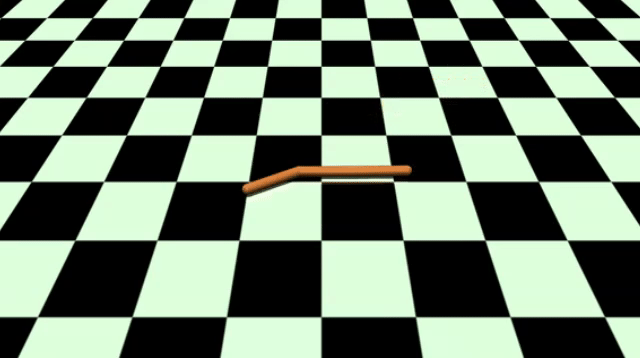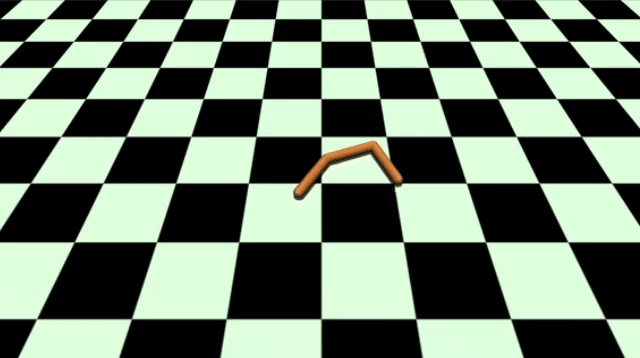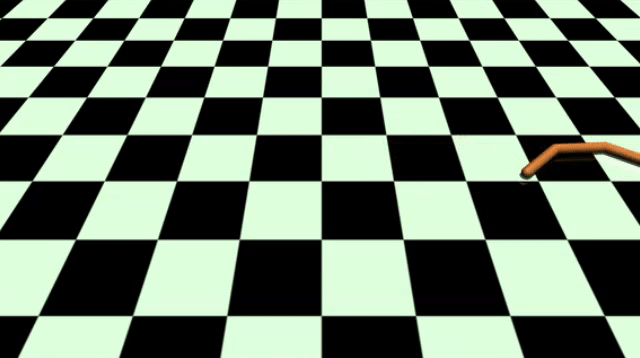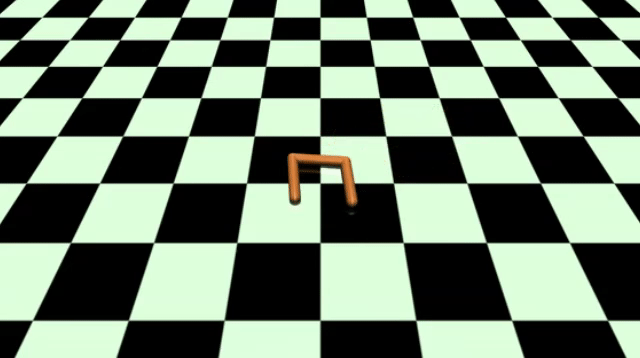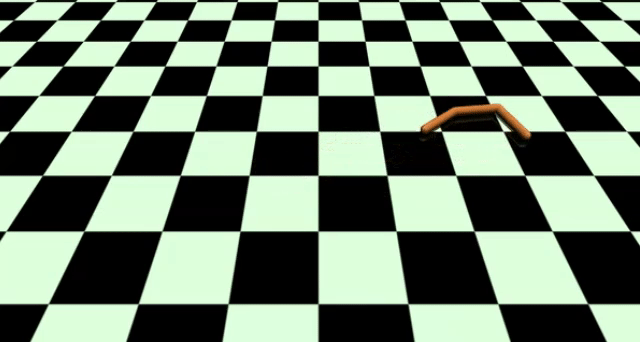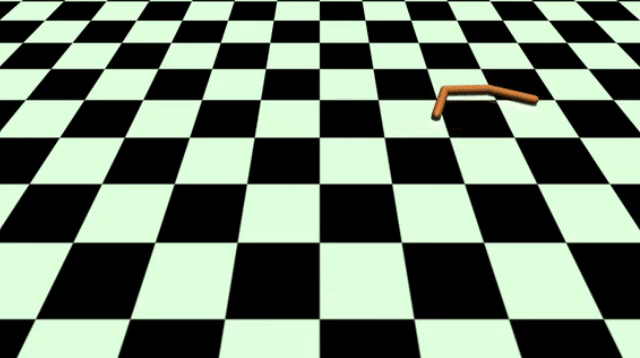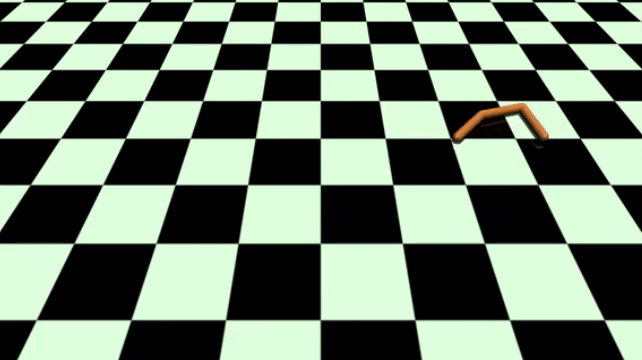Please cite the paper by using the following bibtex.

@article{LiLY22,
author    = {Ke Li and
Guiyu Lai and
Xin Yao},
title     = {Interactive Evolutionary Multi-Objective Optimization via Learning-to-Rank},
journal   = {{IEEE} Trans. Evol. Comput.},
pages     = {1--15},
year      = {2022},
note      = {accepted for publication}
}

•English